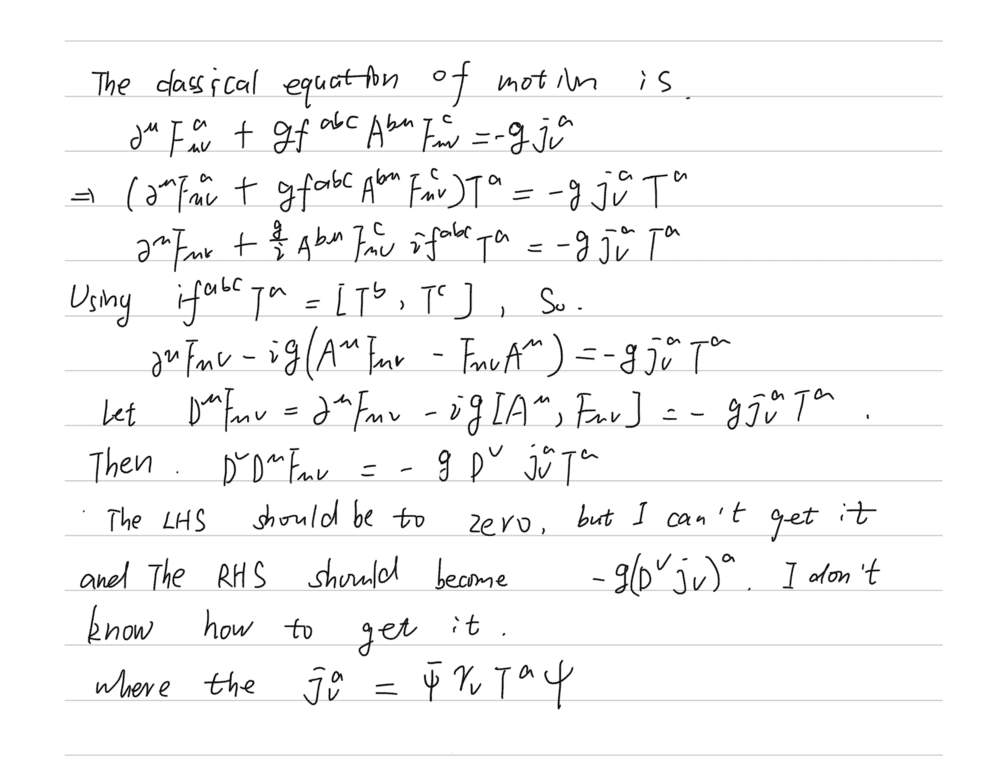# Current conservation for SU (N)

Tian
Homework Statement:
In the "An introduction to Quantum Field Thoery" of Peskin and Schroeder, the equation(15.51) of the chapter 15.3 gives the classical equation of motion, so from this equation to derive the current conservation.
Relevant Equations:
the classical equation of motion for SU(N), please see my picture
Here is my solution$$D^{\mu}F_{\mu\nu} = - j_{\nu},$$ Differentiate this covariantly and anti-symmetrized to obtain $$\frac{1}{2}[D^{\mu}, D^{\nu}]F_{\mu\nu} = D^{\nu}j_{\nu}. \ \ \ \ (1)$$ Now, from the definition of the covariant derivative in the adjoint representation (acting on any matrix-valued field) $$D^{\mu}M \equiv \partial^{\mu}M + [A^{\mu} , M],$$ you can show that $$[D^{\mu} , D^{\nu}]M = [F^{\mu\nu} ,M]$$ Thus, for $M = F_{\mu\nu}$, eq(1) becomes $$D^{\nu}j_{\nu} = \frac{1}{2}[F^{\mu\nu} , F_{\mu\nu}] = 0.$$